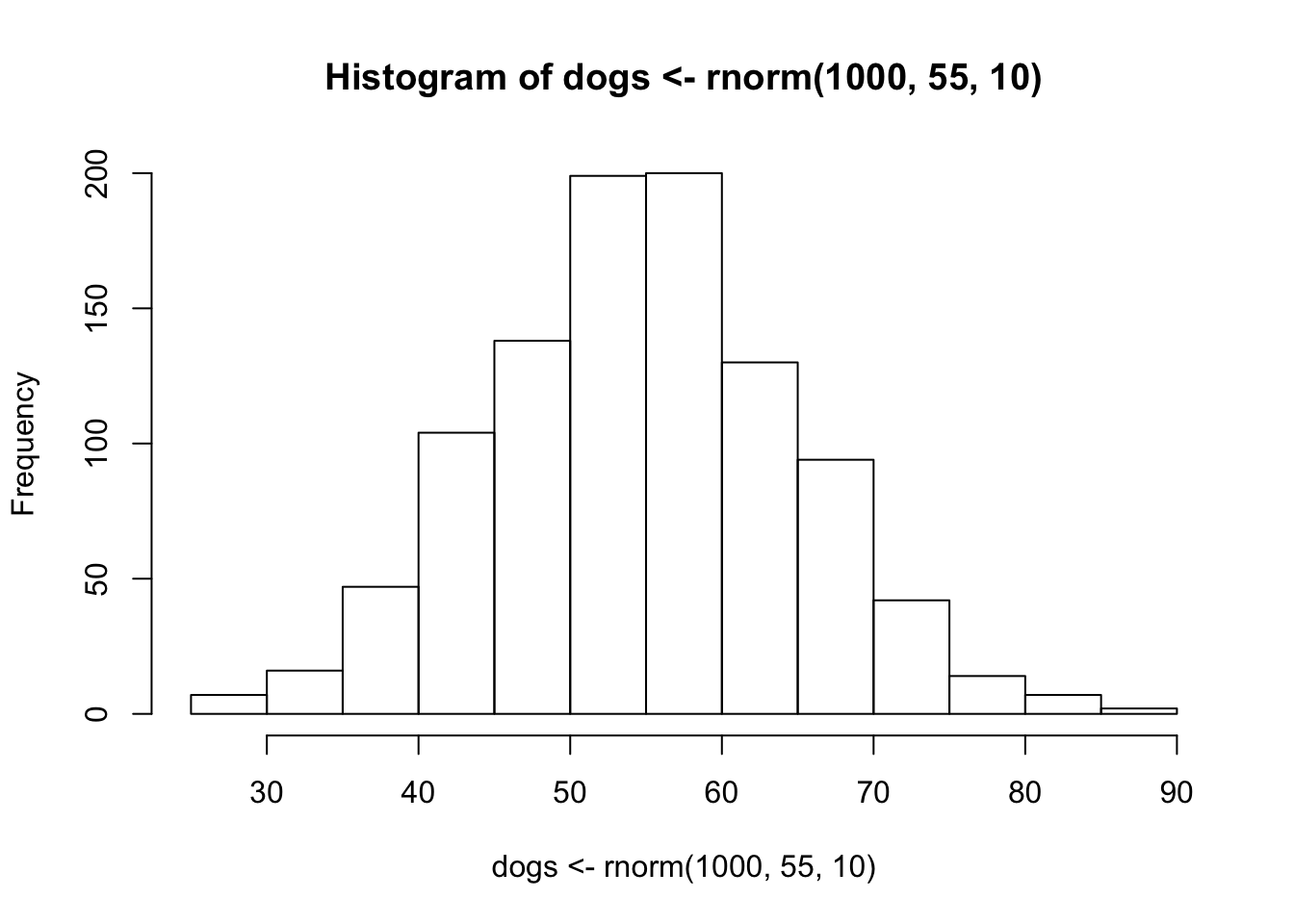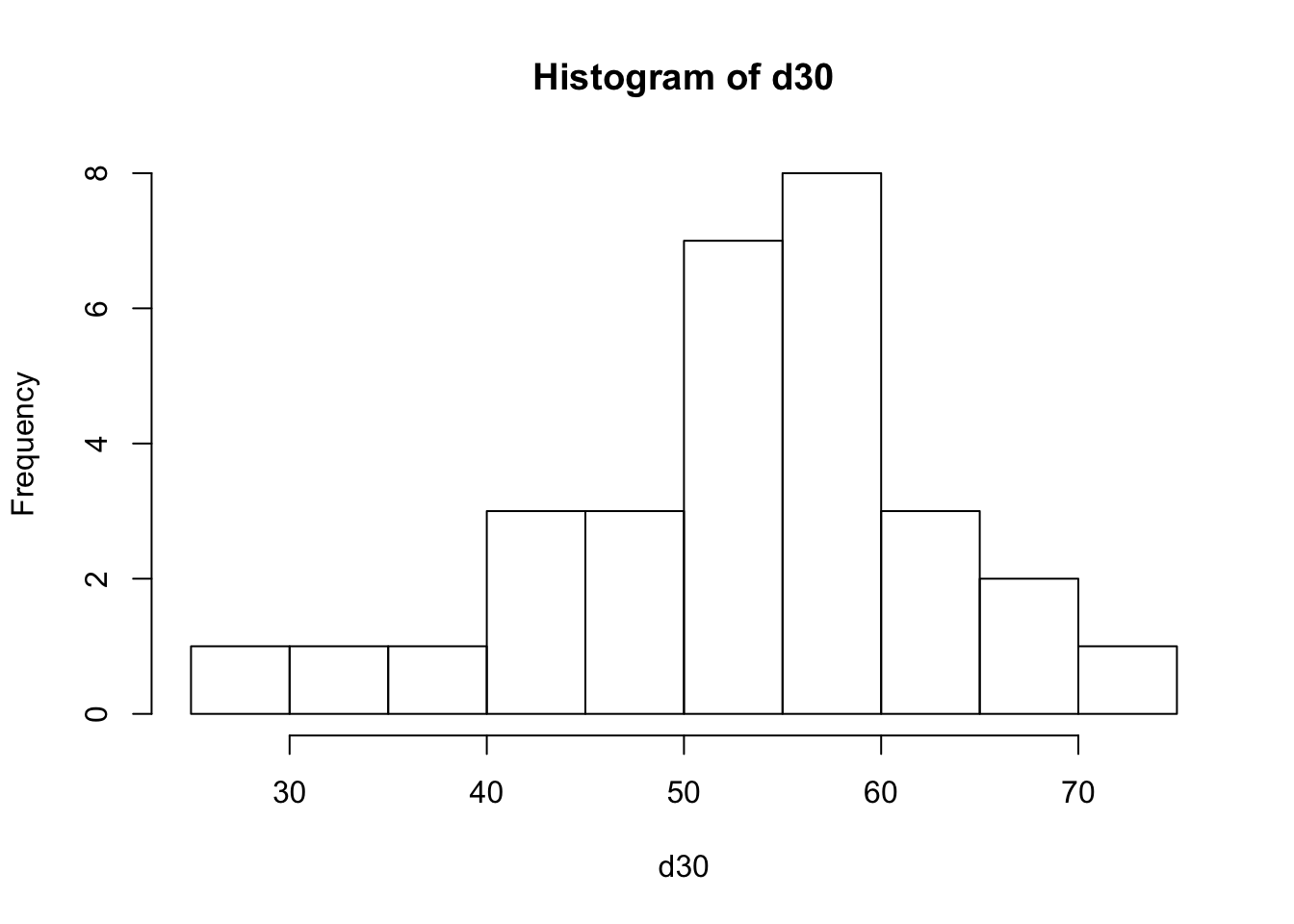Sampling Study Guide

# Sampling

Imagine you have some data on the weight of 1000 golden retriever dogs. The weight of the dogs follows a normal distribution with a mean of 55, and a standard deviation of 10.

The histogram of looks like this. Note where the mean is, and the Y-axis or frequency of the histogram.

hist( dogs <- rnorm(1000, 55, 10))summary(dogs)
##    Min. 1st Qu.  Median    Mean 3rd Qu.    Max.
##   25.91   47.52   54.76   54.70   61.59   86.28

Now you can use a subsample of the dogs data set. Say you want a sample of 30 dogs, to check their mean weight.

d30 <- sample(dogs, 30)

And then get a histogram of the sample size of 30. Note the difference in mean, min and max between the data set for dogs and d30.

summary(d30)
##    Min. 1st Qu.  Median    Mean 3rd Qu.    Max.
##   28.99   47.27   54.44   53.35   59.25   70.42
hist(d30)